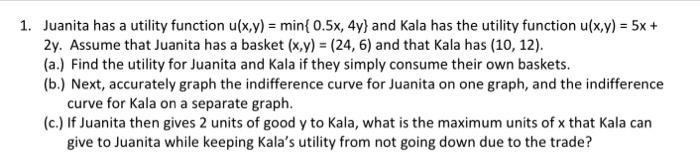1. Juanita has a utility function \( u(x, y)=\min \{0.5 x, 4 y\} \) and Kala has the utility function \( u(x, y)=5 x+ \) \( 2 y \). Assume that Juanita has a basket \( (x, y)=(24,6) \) and that Kala has \( (10,12) \). (a.) Find the utility for Juanita and Kala if they simply consume their own baskets. (b.) Next, accurately graph the indifference curve for Juanita on one graph, and the indifference curve for Kala on a separate graph. (c.) If Juanita then gives 2 units of good \( y \) to Kala, what is the maximum units of \( x \) that Kala can give to Juanita while keeping Kala's utility from not going down due to the trade?

We have an Answer from Expert